Next: 5.1 Basic Assumptions about Up: Math 111 Calculus I Previous: 4.3 The Pythagorean Theorem   Index

# 5. Area

In chapter 2 we calculated the area of the set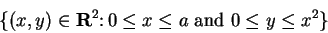where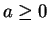, and of the set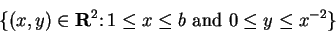where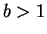.

The technique that was used for making these calculations can be used to find the areas of many other subsets of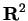. The general procedure we will use for finding the area of a set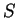will be to find two sequences of polygons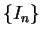and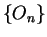such that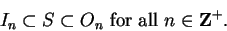We will then have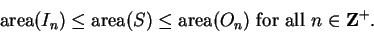(5.1)

We will construct the polygonsand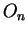so that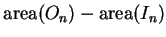is arbitrarily small when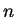large enough, and we will see that then there is a unique number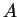such that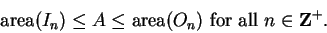(5.2)

We will taketo be the area of.

SubsectionsNext: 5.1 Basic Assumptions about Up: Math 111 Calculus I Previous: 4.3 The Pythagorean Theorem   Index
Ray Mayer 2007-09-07